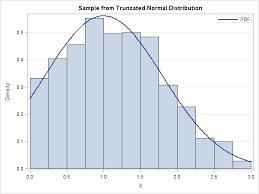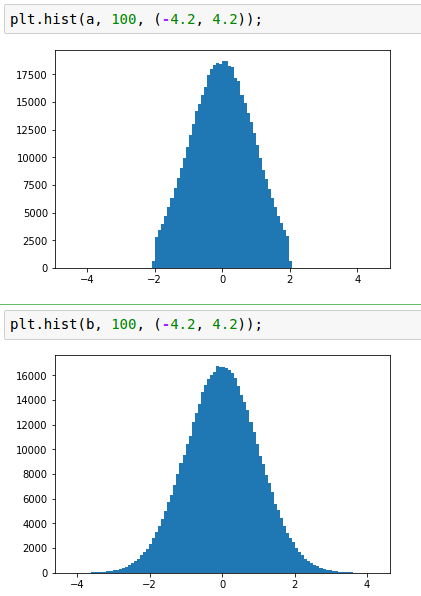2 views

tf.random_normal(shape, mean=0.0, stddev=1.0, dtype=tf.float32, seed=None, name=None)

outputs random values from a normal distribution.

tf.truncated_normal(shape, mean=0.0, stddev=1.0, dtype=tf.float32, seed=None, name=None)

outputs random values from a truncated normal distribution.

I tried googling 'truncated normal distribution'. But didn't understand much.

by (33.1k points)

Truncated normal distribution:

The values generated from this function follows a normal distribution with specified mean and standard deviation, except that values whose magnitude is more than 2 standard deviations from the mean are dropped and re-picked.It is easy to understand the difference by plotting the graph for yourself (%magic is used because I use jupyter notebook):

Code to plot graph:

import tensorflow as tf

import matplotlib.pyplot as plt

%matplotlib inline

n = 500000

A = tf.truncated_normal((n,))

B = tf.random_normal((n,))

with tf.Session() as sess:

a, b = sess.run([A, B])

plt.hist(a, 100, (-4.2, 4.2)); plt.hist(b, 100, (-4.2, 4.2));The raeson for using truncated normal is to overcome saturation of time functions like sigmoid.